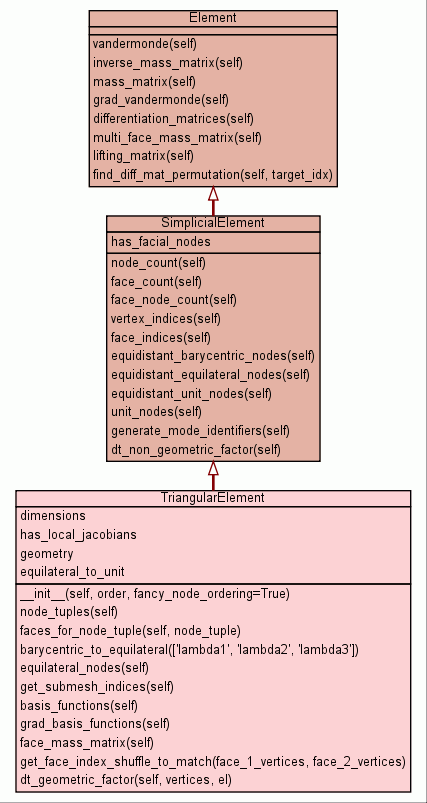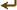[frames] | no frames]

# Class TriangularElement

source codeAn arbitrary-order triangular finite element.

# Coordinate systems used:

unit coordinates (r,s):

```
```

C |\ | \ | O | \ | \ A-----B

Points in unit coordinates:

```   O = (0,0)
A = (-1,-1)
B = (1,-1)
C = (-1,1)
```

equilateral coordinates (x,y):

```       C
/ \
/   \
/     \
/   O   \
/         \
A-----------B
```

Points in equilateral coordinates:

```   O = (0,0)
A = (-1,-1/sqrt(3))
B = (1,-1/sqrt(3))
C = (0,2/sqrt(3))
```

When global vertices are passed in, they are mapped to the reference vertices A, B, C in order.

Faces are always ordered AB, BC, AC.

 Nested Classes
geometry
 Instance Methods

 __init__(self, order, fancy_node_ordering=True) x.__init__(...) initializes x; see x.__class__.__doc__ for signature source code

 node_tuples(self) Generate tuples enumerating the node indices present in this element. source code

 faces_for_node_tuple(self, node_tuple) Return the list of face indices of faces on which the node represented by `node_tuple` lies. source code

 equilateral_nodes(self) Generate warped nodes in equilateral coordinates (x,y). source code

 get_submesh_indices(self) Return a list of tuples of indices into the node list that generate a tesselation of the reference element. source code

 basis_functions(self) Get a sequence of functions that form a basis of the approximation space. source code

 grad_basis_functions(self) Get the gradient functions of the basis_functions(), in the same order. source code

 face_mass_matrix(self) source code

 dt_geometric_factor(self, vertices, el) source code

Inherited from `SimplicialElement`: `dt_non_geometric_factor`, `equidistant_barycentric_nodes`, `equidistant_equilateral_nodes`, `equidistant_unit_nodes`, `face_count`, `face_indices`, `face_node_count`, `generate_mode_identifiers`, `node_count`, `unit_nodes`, `vertex_indices`

Inherited from `Element`: `differentiation_matrices`, `find_diff_mat_permutation`, `grad_vandermonde`, `inverse_mass_matrix`, `lifting_matrix`, `mass_matrix`, `multi_face_mass_matrix`, `vandermonde`

Inherited from `object`: `__delattr__`, `__getattribute__`, `__hash__`, `__new__`, `__reduce__`, `__reduce_ex__`, `__repr__`, `__setattr__`, `__str__`

 Static Methods

 barycentric_to_equilateral((lambda1, lambda2, lambda3)) Return the equilateral (x,y) coordinate corresponding to the barycentric coordinates (lambda1..lambdaN). source code

 get_face_index_shuffle_to_match(face_1_vertices, face_2_vertices) source code
 Class Variables
dimensions = `2`
has_local_jacobians = `False`
equilateral_to_unit = `AffineMap(numpy.array([[1,-1/ sqrt(3)], ...`
 Properties

Inherited from `SimplicialElement`: `has_facial_nodes`

Inherited from `object`: `__class__`

 Method Details

### __init__(self, order, fancy_node_ordering=True)(Constructor)

source code

x.__init__(...) initializes x; see x.__class__.__doc__ for signature

Overrides: object.__init__
(inherited documentation)

### node_tuples(self)

source code

Generate tuples enumerating the node indices present in this element. Each tuple has a length equal to the dimension of the element. The tuples constituents are non-negative integers whose sum is less than or equal to the order of the element.

The order in which these nodes are generated dictates the local node numbering.

Decorators:
• `@memoize_method`

### get_submesh_indices(self)

source code

Return a list of tuples of indices into the node list that generate a tesselation of the reference element.

Decorators:
• `@memoize_method`

### basis_functions(self)

source code

Get a sequence of functions that form a basis of the approximation space.

The approximation space is spanned by the polynomials::

``` r**i * s**j for i+j <= N
```
Decorators:
• `@memoize_method`
Overrides: Element.basis_functions

### grad_basis_functions(self)

source code

Get the gradient functions of the basis_functions(), in the same order.

Overrides: Element.grad_basis_functions

### face_mass_matrix(self)

source code
Decorators:
• `@memoize_method`

 Class Variable Details

### equilateral_to_unit

Value:
 ```AffineMap(numpy.array([[1,-1/ sqrt(3)], [0, 2/ sqrt(3)]]), numpy.array([-1/ 3,-1/ 3])) ```

 Generated by Epydoc 3.0.1 on Sat Aug 29 14:33:26 2009 http://epydoc.sourceforge.net